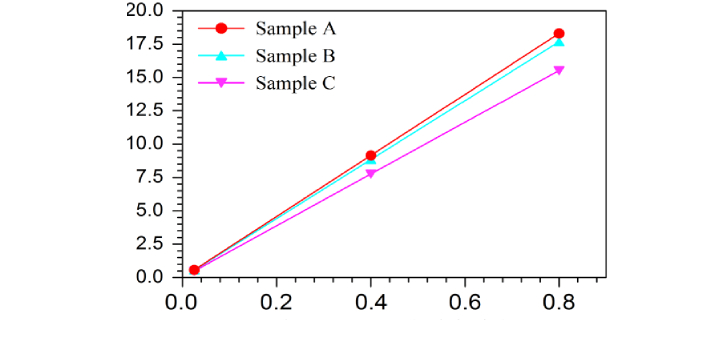What is the difference between Rate and Ratio? - ProProfs Discuss+ Ask Question

# What is the difference between Rate and Ratio?

Asked by B. Wright, Last updated: Nov 26, 2020

###Request 0FollowShareAnswer AnonymouslyAnswer LaterCopy LinkHoward Reyes, Science Teacher, California

Rate and Ratio are two terms you are more likely to encounter in subjects like mathematics, business, etc. You will actually find them interesting if you understand what they stand for and how they are calculated. The term 'rate' can be used in various ways. First of all, you can use it to determine the proportional relationship between one amount and another. For example: with the recent development in the department, the company can now produce machines at the rate of 20 a year.It can also be used to describe the speed with which something is moving. For example The motorcycle is moving at one hell of a rate. It can also be used to refer to the price of the thing. Ratio, on the other hand, is used to determine the relationship between two or more similar items in terms of quantity, amount, and size. Unlike rate, which defines the relationship between two items, Ratio can be used for more than two items.W. Kaye, Ex-Marine, Post Graduate, Chapel HillRate is the relationship between two measurements that have discrete units. The quantity or unit, where a distinct thing is unspecified, is generally the rate per unit time. The most typical kind of standard is time, such as heart rate and speed. The ratio is the connection of the two numbers which have the same type. It may pertain to spoonful's, units, students, persons, and objects.Rate relates to a fixed quantity between two things, while a ratio is a relationship between lots of things. Rate and ratio are significant in explaining the equivalence from one and the other. A rate cannot be one if the ratio does not exist, as the rate is used for measurements. Both are used in mathematics to determine a matter's proportion or value.Search for Google imagesSelect a recommended image
Upload from your computerCancelSearch for Google imagesSelect a recommended image
Upload from your computerCancelSearch for Google imagesSelect a recommended image
Upload from your computerCancel